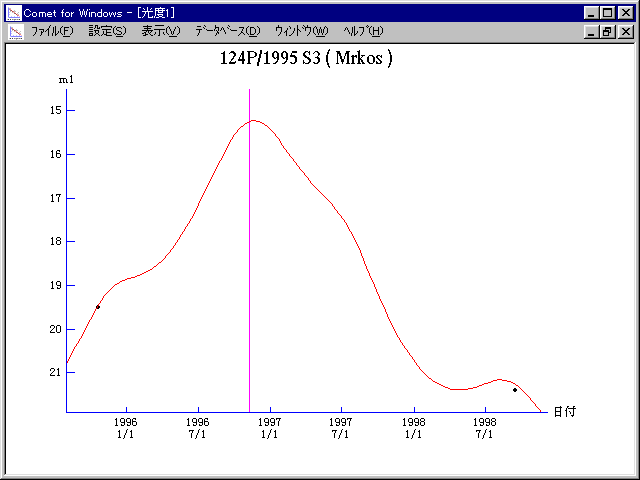# \$B%`%k%3%9WB@1(B

124P/Mrkos (1996)

 English version Home page Updated on December 22, 1998###\$B%W%m%U%#!<%k(B

 \$BId9f(B 124P/1995 S3 \$B8!=PF|(B 1995\$BG/(B9\$B7n(B20\$BF|(B, 9\$B7n(B28\$BF|(B \$B8!=P8wEY(B 20.2\$BEy(B \$B8!=P C. W. Hergenrother, W. Offutt (Kitt Peak)

###\$B50F;MWAG(B

```   The following improved orbital elements by Kenji Muraoka, are
from 65 observations  1991 to 1998, perturbations by 9 Plantes,
Moon and 5 minor planets were taken into account.
The mean residual is +/- 0.75 arc seconds.

Epoch  =  1996 Nov. 13.0  TT       JDT = 2450400.5
T  =  1996 Nov.  9.07059       +/- 0.00039 (m.e.) TT
Peri. =  180.52130                +/- 0.00030
Node  =    1.65283                +/- 0.00002   (2000.0)
Incl. =   31.47082                +/- 0.00005
q  =    1.4128940              +/- 0.0000018 AU
e  =    0.5542498              +/- 0.0000003
a  =    3.1696989              +/- 0.0000015 AU
n  =    0.17465340             +/- 0.00000012
P  =    5.643                  +/- 0.0000039  years
```

###\$B@1?^(B1995\$BG/(B 9\$B7n(B10\$BF|!A(B1996\$BG/(B 9\$B7n(B24\$BF|(B1996\$BG/(B 8\$B7n(B15\$BF|!A(B1997\$BG/(B 1\$B7n(B 2\$BF|(B1996\$BG/(B11\$B7n(B23\$BF|!A(B1997\$BG/(B 9\$B7n(B19\$BF|(B

###\$B8wEYJQ2=(B

```        m1 = 12.0 + 5 log\$B&\$(B + 10.0 log r
```##### \$B50F;MWAG\$OB<2,7r<#;a\$N7W;;\$K\$h\$k\$b\$N\$G\$9!#(B \$B@1?^\$O(B StellaNavigator Ver.2.0 for Windows (\$B%"%9%H%m%"!<%D(B \$BJTCx(B / \$B%"%9%-!<=PHG6I4)(B) \$B\$G:n@.\$7\$?\$b\$N\$G\$9!#(B \$B8wEY%0%i%U\$O(BComet for Windows\$B\$G:n@.\$7\$?\$b\$N\$G\$9!#(B